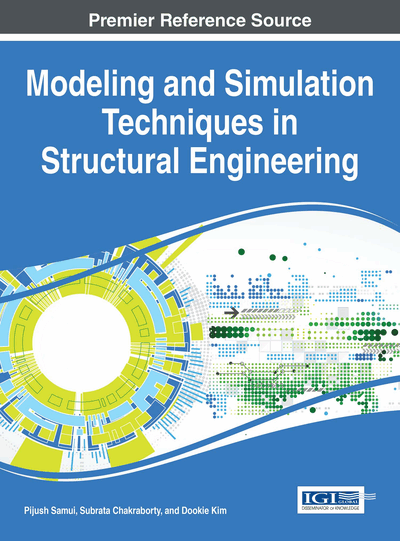# Polynomial Correlated Function Expansion

Souvik Chakraborty (Indian Institute of Technology Roorkee, India) and Rajib Chowdhury (Indian Institute of Technology Roorkee, India)
DOI: 10.4018/978-1-5225-0588-4.ch012

## Abstract

Uncertainty quantification of civil engineering structures are often time consuming. Hence, one has to rely on equivalent models (EM) that represent the original functions with a specific level of accuracy. In this chapter, a fully equivalent operational model, referred as, Polynomial Correlated Function Expansion (PCFE) has been discussed. PCFE facilitates a systematic mapping between the input and output by expressing the output as a ranked order of component functions, with higher order component functions representing higher-order cooperative effect. The component functions are expressed in terms of extended bases and the unknown coefficients associated with the bases are determined by employing homotopy algorithm (HA). HA determines the unknown coefficients by minimizing the least-squared error and imposing an additional criteria defined as an objective function. Implementation of PCFE has been illustrated with two large scale problems. Results obtained have been compared with other popular techniques. For both the problems, PCFE outperforms popular EMs available in literature.
Chapter Preview
Top

## Introduction

Uncertainties in load and system properties play a significant role in reliability analysis of structural/mechanical systems. The subject of uncertainty quantification has evolved over the last few decades to deal with various uncertainties. A well-developed body of literature (Ditlevsen & Madsen, 1996; Melchers, 1999) now exists which documents the status of this subject. However, most of the available methods for uncertainty quantification are suitable for simple academic problems involving very few random variables. Difficulties in the analysis of structures with parameter uncertainties primarily arise due to nonlinear variation of the response variables to the stochastic system parameters; this being true even when structures are idealized to display linear material and deformation characteristics. The problem is further enhanced when nonlinear structural behavior is included in the analysis.

Unfortunately, stochastic methods that are utilized for uncertainty quantification are inaccurate and/or computationally inefficient when:

• 1.

The input-output relationship is highly nonlinear;

• 2.

The number of input random variables or fields is large; and

• 3.

There are large statistical variations in input.

For example, the most common approach for uncertainty quantification based on Taylor’s series expansion are not adequate for highly nonlinear problems. Simulation methods, which are usually employed for obtaining benchmark results, are not computationally efficient, and are not suitable to being embedded in optimization processes. Hence, new stochastic methods are needed to achieve greater accuracy and/or better efficiency than traditional methods.

In order to alleviate the computational issue associated with uncertainty quantification, various equivalent models (EM) has been developed. EM are utilized to generate surrogates for original function based on actual simulation at significantly reduces number of sample points. Parameters/unknown coefficients of the EM are determined by minimizing the difference between the two models (i.e., original and equivalent) in some statistical sense. Once the unknown coefficients are determined, uncertainty of the response is quantified by performing simulations on the generated equivalent model. Application of EM can be found in design optimization (Dubourg, Sudret, & Bourinet, 2011), design space exploration (Adhikari, Chowdhury, & Friswell, 2011), sensitivity analysis (Sobol, 1993) etc.

The most popular method for constructing EM is the response surface method (RSM) based on least-square regression (LSR) (Wu, 2009). In RSM, an EM is constructed by utilizing the second order polynomials. The unknown coefficients are determined by minimizing the least squared error. Number of modifications to the conventional RSM (such as RSM based on least-angle regression, high-order stochastic response surface method (HO-SRSM) (Gavin & Yau, 2008) and RSM based on moving least-square regression (Goswami, Chakraborty, & Ghosh, 2013)), have been suggested by the researchers. However, all the variants of RSM yield satisfactory results only for problems having a lower degree of nonlinearity. Another method that is quite popular among researchers is the Kriging or Gaussian process regression (GPR) (Echard, Gayton, Lemaire, & Relun, 2013). In GPR, the interpolated values are modelled by Gaussian process governed by prior covariances, as opposed to a piece-polynomial spline to optimize smoothness of the predicted values. The basic idea of GPR is to predict the value of a function at a given point by computing a weighted average of the known values of the function in the neighbourhood of the point. Although GPR is mathematically closely related to the conventional regression analysis, the basic difference resides in the fact that while conventional regression is based on multiple observations of a multivariate data set, GPR is used for estimation of a single realization. However, GPR requires significant number of sample points (actual experiments/FE analysis), rendering the process inefficient for high dimensional problems.

## Complete Chapter List

Search this Book:
Reset